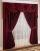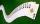# Fraction calculator

The calculator performs basic and advanced operations with fractions, expressions with fractions combined with integers, decimals, and mixed numbers. It also shows detailed step-by-step information about the fraction calculation procedure. Solve problems with two, three, or more fractions and numbers in one expression.

## Result:

### 73/15 + 912/15 = 17/1 = 17

Spelled result in words is seventeen.

### How do you solve fractions step by step?

1. Conversion a mixed number 7 3/15 to a improper fraction: 7 3/15 = 7 3/15 = 7 · 15 + 3/15 = 105 + 3/15 = 108/15

To find new numerator:
a) Multiply the whole number 7 by the denominator 15. Whole number 7 equally 7 * 15/15 = 105/15
b) Add the answer from previous step 105 to the numerator 3. New numerator is 105 + 3 = 108
c) Write a previous answer (new numerator 108) over the denominator 15.

Seven and three fifteenths is one hundred eight fifteenths
2. Conversion a mixed number 9 12/15 to a improper fraction: 9 12/15 = 9 12/15 = 9 · 15 + 12/15 = 135 + 12/15 = 147/15

To find new numerator:
a) Multiply the whole number 9 by the denominator 15. Whole number 9 equally 9 * 15/15 = 135/15
b) Add the answer from previous step 135 to the numerator 12. New numerator is 135 + 12 = 147
c) Write a previous answer (new numerator 147) over the denominator 15.

Nine and twelve fifteenths is one hundred forty-seven fifteenths
3. Add: 108/15 + 147/15 = 108 + 147/15 = 255/15 = 15 · 17/15 · 1 = 17
For adding, subtracting, and comparing fractions, it is suitable to adjust both fractions to a common (equal, identical) denominator. The common denominator you can calculate as the least common multiple of both denominators - LCM(15, 15) = 15. In practice, it is enough to find the common denominator (not necessarily the lowest) by multiplying the denominators: 15 × 15 = 225. In the next intermediate step, , cancel by a common factor of 15 gives 17/1.
In words - one hundred eight fifteenths plus one hundred forty-seven fifteenths = seventeen.

#### Rules for expressions with fractions:

Fractions - use the slash “/” between the numerator and denominator, i.e., for five-hundredths, enter 5/100. If you are using mixed numbers, be sure to leave a single space between the whole and fraction part.
The slash separates the numerator (number above a fraction line) and denominator (number below).

Mixed numerals (mixed fractions or mixed numbers) write as non-zero integer separated by one space and fraction i.e., 1 2/3 (having the same sign). An example of a negative mixed fraction: -5 1/2.
Because slash is both signs for fraction line and division, we recommended use colon (:) as the operator of division fractions i.e., 1/2 : 3.

Decimals (decimal numbers) enter with a decimal point . and they are automatically converted to fractions - i.e. 1.45.

The colon : and slash / is the symbol of division. Can be used to divide mixed numbers 1 2/3 : 4 3/8 or can be used for write complex fractions i.e. 1/2 : 1/3.
An asterisk * or × is the symbol for multiplication.
Plus + is addition, minus sign - is subtraction and ()[] is mathematical parentheses.
The exponentiation/power symbol is ^ - for example: (7/8-4/5)^2 = (7/8-4/5)2

#### Examples:

subtracting fractions: 2/3 - 1/2
multiplying fractions: 7/8 * 3/9
dividing Fractions: 1/2 : 3/4
exponentiation of fraction: 3/5^3
fractional exponents: 16 ^ 1/2
adding fractions and mixed numbers: 8/5 + 6 2/7
dividing integer and fraction: 5 ÷ 1/2
complex fractions: 5/8 : 2 2/3
decimal to fraction: 0.625
Fraction to Decimal: 1/4
Fraction to Percent: 1/8 %
comparing fractions: 1/4 2/3
multiplying a fraction by a whole number: 6 * 3/4
square root of a fraction: sqrt(1/16)
reducing or simplifying the fraction (simplification) - dividing the numerator and denominator of a fraction by the same non-zero number - equivalent fraction: 4/22
expression with brackets: 1/3 * (1/2 - 3 3/8)
compound fraction: 3/4 of 5/7
fractions multiple: 2/3 of 3/5
divide to find the quotient: 3/5 ÷ 2/3

The calculator follows well-known rules for order of operations. The most common mnemonics for remembering this order of operations are:
PEMDAS - Parentheses, Exponents, Multiplication, Division, Addition, Subtraction.
BEDMAS - Brackets, Exponents, Division, Multiplication, Addition, Subtraction
BODMAS - Brackets, Of or Order, Division, Multiplication, Addition, Subtraction.
GEMDAS - Grouping Symbols - brackets (){}, Exponents, Multiplication, Division, Addition, Subtraction.
Be careful, always do multiplication and division before addition and subtraction. Some operators (+ and -) and (* and /) has the same priority and then must evaluate from left to right.

## Fractions in word problems:To three-eighths of one third, we add five quarters of one half and multiply the sum by four. How much will we get?
• Weigh in totalI put 3/5 kg of grapes into a box which is 1/4kg in weight. How many kilograms do the grapes and the box weigh in total?
• Math homeworkIt took Jose two-thirds of an hour to complete his math homework on Monday, three-fourths of an hour on Tuesday, any two- fifths of an hour on Wednesday. How many hours did it take Jose to complete his homework altogether?
• Evaluate fractionsThe difference  of 5 1/2 and 2/3 is added to the product of 5/6 and 1/2
• Interior designerTo make draperies an interior designer needs 11 1/4 yards of material for the den and 8 1/2 yards for the living room. If material comes only in 20 yard bolts, how much will be left over after completing both sets of draperies?
• Area and perimeter 2Find the area and the perimeter of a rectangle of length 45 1/2 cm and breadth 16 2/3 cm.
• An orchardDuring a visit to an orchard, Greg picked 3/5 of a bag of delicious golden apples, 4/5 of a bag of Macintosh apples, 2/5 of a bag of Cortland apples, 1/5 of a bag of Bartlett pears, and 4/5 of a bag of Bosch pears. How many bags of fruit to Greg pick in tAdd two mixed fractions: 2 4/6 + 1 3/6The first team member in a 926-person relay race must run 2 1/4 laps, the second team member must run 1 1/2 laps, and the third team member must run 3 1/4 laps. How many laps in all must each team run?3 3/4 + 2 3/5 + 5 1/2 Show your solution.Ali bought 5/6 litre of milk. He drank 1/2 litre and his brother drank 1/6 litre. How much litre of milk left?Kara has 2 times more cards than Dana, Dana has 4× less than Mary. Together they have 728 cards. How many cards has each of them?The sum of two rational numbers is (-2). If one of them is 3/5, find the other.